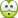# Prove the following identity [Einstein notation]

## Homework Statement

[/B]
Prove the following identity:

$$\vec{\nabla}(\vec{A} \cdot \vec{B}) = (\vec{A} \cdot \vec{\nabla})\vec{B} + (\vec{B} \cdot \vec{\nabla})\vec{A} + \vec{A} \times (\vec{\nabla} \times \vec{B}) + \vec{B} \times (\vec{\nabla} \times \vec{A})$$

## Homework Equations

Kronecker's delta, levi-civita tensor

## The Attempt at a Solution

[/B]
My solution consisted of simply solving the RHS by decomposition. I wrote $$\vec{A} = A_{1}A_{\hat{x}} + A_{2}A_{\hat{y}} + A_{3}A_{\hat{z}}$$ likewise with B. I manually solved for each term in RHS and I did get the correct result which was LHS. My problem is that there is probably a more efficient way of doing this, perhaps by incorporating Einstein notation and solve LHS immediately without having to look at RHS.

How should I do this?

I tried $$\vec{\nabla}(\vec{A} \cdot \vec{B}) = \partial_{x_{i}}A_{i}B_{i} = \frac{\partial A_{i}}{\partial_{x_{i}}}B_{i} + A_{i} \frac{\partial B_{i}}{\partial_{x_{i}}}$$ but then I got stuck. How to continiue? I'm not sure how all those $$\times$$ appear using einstein notation.

nrqed
Homework Helper
Gold Member

## Homework Statement

[/B]
Prove the following identity:

$$\vec{\nabla}(\vec{A} \cdot \vec{B}) = (\vec{A} \cdot \vec{\nabla})\vec{B} + (\vec{B} \cdot \vec{\nabla})\vec{A} + \vec{A} \times (\vec{\nabla} \times \vec{B}) + \vec{B} \times (\vec{\nabla} \times \vec{A})$$

## Homework Equations

Kronecker's delta, levi-civita tensor

## The Attempt at a Solution

[/B]
My solution consisted of simply solving the RHS by decomposition. I wrote $$\vec{A} = A_{1}A_{\hat{x}} + A_{2}A_{\hat{y}} + A_{3}A_{\hat{z}}$$ likewise with B. I manually solved for each term in RHS and I did get the correct result which was LHS. My problem is that there is probably a more efficient way of doing this, perhaps by incorporating Einstein notation and solve LHS immediately without having to look at RHS.

How should I do this?

I tried $$\vec{\nabla}(\vec{A} \cdot \vec{B}) = \partial_{x_{i}}A_{i}B_{i} = \frac{\partial A_{i}}{\partial_{x_{i}}}B_{i} + A_{i} \frac{\partial B_{i}}{\partial_{x_{i}}}$$ but then I got stuck. How to continiue? I'm not sure how all those $$\times$$ appear using einstein notation.
You should not have the same index (here the "i") used three times in an expression, this is a big no-no. Also notice that your left side is a vector an your right side is a scalar, so that cannot be right. What you need is

$$\hat{e}_j ~\partial_j (A_i B_i )$$
where by ##\partial_j## I mean
$$\partial_j \equiv \frac{\partial}{\partial x_j}$$.

•BvU
BvU
Homework Helper
Einstein notation is just a shorthand -- doesn't help you derive something. Might even confuse some folks ...This any use ? (where you may need the liberty to read ##(a\cdot\nabla)## as ##(\nabla\cdot a)## by virtue of ##a\cdot b = b \cdot a ## ... )

Must admit I got stuck with factors of 2, thoughnrqed
Einstein notation is just a shorthand -- doesn't help you derive something. Might even confuse some folks ...Must admit I got stuck with factors of 2, though•